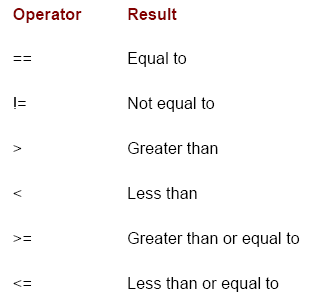# Programming Tutorials

## Relational Operators in Java

By: Jagan Printer Friendly Format

The relational operators determine the relationship that one operand has to the other. Specifically, they determine equality and ordering. The relational operators are shown here:

The outcome of these operations is a boolean value. The relational operators are most frequently used in the expressions that control the if statement and the various loop statements.

Any type in Java, including integers, floating-point numbers, characters, and Booleans can be compared using the equality test, ==, and the inequality test, !=. Notice that in Java (as in C and C++) equality is denoted with two equal signs, not one. (Remember: a single equal sign is the assignment operator.) Only numeric types can be compared using the ordering operators. That is, only integer, floating-point, and character operands may be compared to see which is greater or less than the other. As stated, the result produced by a relational operator is a boolean value.For example, the following code fragment is perfectly valid:

int a = 4;
int b = 1;
boolean c = a < b;

In this case, the result of a<b (which is false) is stored in c.
If you are coming from a C/C++ background, please note the following. In C/C++, these types of statements are very common:

int done;
// ...
if(!done) ... // Valid in C/C++
if(done) ... // but not in Java.
In Java, these statements must be written like this:
if(done == 0)) ... // This is Java-style.
if(done != 0) ...

The reason is that Java does not define true and false in the same way as C/C++. In C/C++, true is any nonzero value and false is zero. In Java, true and false are nonnumeric values which do not relate to zero or nonzero. Therefore, to test for zero or nonzero, you must explicitly employ one or more of the relational operators.

 Most Viewed Articles (in Java ) Latest Articles (in Java)

Comment on this tutorial